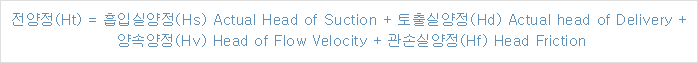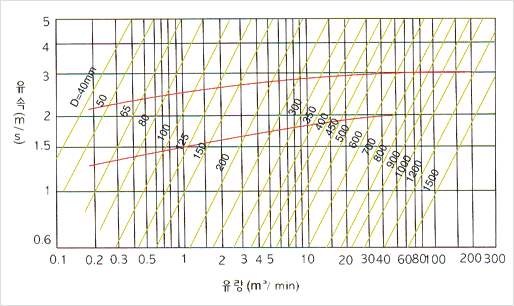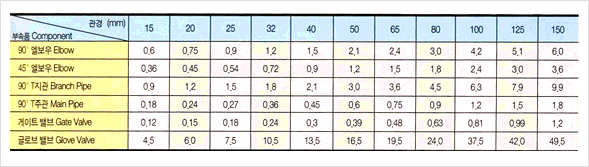Pump Head is the vertical height to which pump can take up water and the total head is calculated by adding
converted vertical height of the losses according to the length and diameter of pipe to the actual vertica| height.
However, it is not so easy to convert the head into vertical height, because it is changed according to the |ength,
diameter, quantity and condition of pipe and the water temperature ln case the loss head is calculated too much,
it can be an unnecessarily high-pressure pump and also has a risk of overloading despite more water comes out
and it is actually run with the lower pressur. ln case the loss head ls calculated too less, water actually does not
come out and comes out less with higher pressure.
Therefore, it is very important to calculate loss head properly.Hs Actual Head of Suction (m) : The vertical height from the center line of pump suction inlet to the
suction water level.Hd Actural Head of Delivery (m) : The verical height from te center line of pump suction inlet to the
highest water level of storage tankHv Head of Flow Velocity (m) : This is cuased from the difference of suction and discharge caliber.
If both caliber. If bth caliber is equal, the value is zero, but even though                                                               there is difference,the value is small so that w can ignore it.Hf Head of Friction Loss(m) :  There are many methods to cahulate friction loss, the most ordinary way
as Darcy formu|a is as below.Calculation Method of Friction Loss Head(Darcy Formula)f : Friction loss coefficient of pipe(0.020+0.0005/D) New Pipe, Old pipe=0.04
D : Diameter of pipe(m)
L : Length of pipe(m)
V : Flow velocity of pipe(m/sec)
g : Gravitational acceleration (9.81 m/sec2)Flow rate and flow velocityFriction Loss of piping componenis (convered to the length of straight type horizontal pipe)(unit:m)